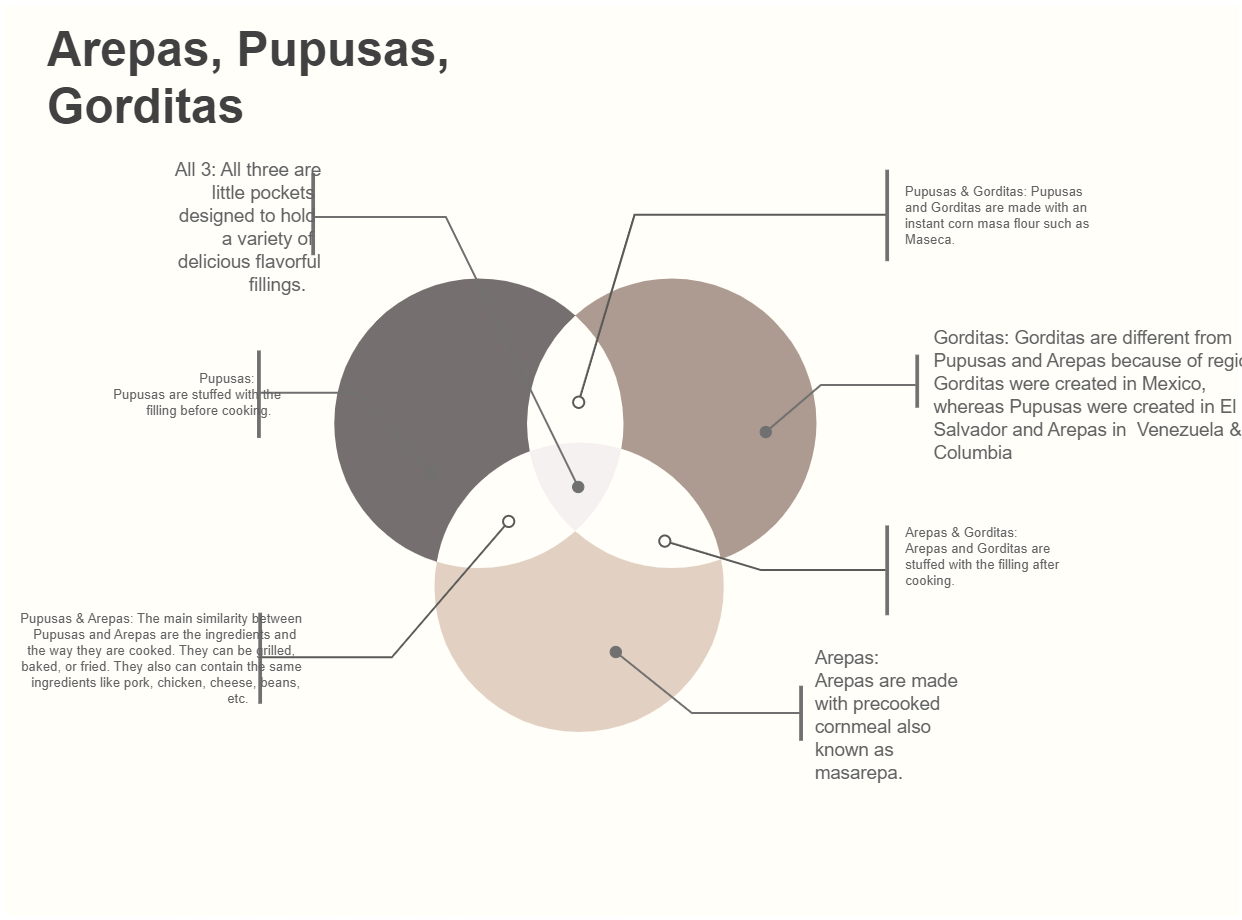Template Community / Venn Diagram Example

# Venn Diagram Example

Share
Duplicate19
2
Report
Publish time：11-11-2021
A Venn diagram example depicts the relationship between entities that have something common to share. It is drawn by making circles. The circles that overlap each other have something in common. While the circles that do not overlap have nothing in common. A Venn diagram example visually depicts similarities and differences by juxtaposing both. In order to draw a Venn diagram, first, draw circles and make sure each circle overlaps at least one circle. Write the differences and similarities of the concept in the circle and label the diagram. Venn diagrams examples are mainly used by teachers, mathematicians to explain complex concepts to the students in an easier way.
Creator
a
alexiskblairPost
Recommended TemplatesPublish
Your
Diagram